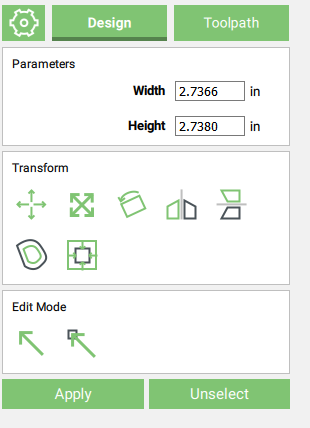# CC 451 Circle resizing on its own

William / Crew.

CC 451.

Draw a 2.73 circle
draw a rectangle inside that circle
align rectangle to left inside edge of circle
use boolean subtract to make circle look like this

circle is now width of 2.7366 and height of 2.7380What gives?

Example of a 3" circle

Step 1

Step 2

Step 3

Are we building a Steinway?

not sure why you question the accuracy I need but it is what I need

for the record… does exactly the same thing in VCARVE PRO - however i can at least go back to it’s diameter and change it from 2.728 height and 2.73 width to 2.73 uniformly. CC does not function this way. When I go back to edit said diameter, when I enter height and width as 2.73, hit apply, then some other arbitrary number is now in both cells.

Odd behavior… would think a set diameter is a set diameter. How does conjoining a rectangle affect the diameter of the previously set circle?

Shouldn’t have any effect on the height; however, you do lose a very small amount in width. Did you look at the size of the circle after drawing it and before the boolean operation? It could be that boolean operations convert the circle to a polygon so it’s like measuring a hex nut across the flats vs. across the points.

Significant figures…and units…

That should be correct — once that is removed it is no longer a circle — you could use Pythagorean’s Theorem to calculate how much is removed — here’s a visual:

Note that if you rotate the shape 45 degrees it then reflects the original measurement:

If I’m misunderstanding the difficulty, please work up an explanation which shows a flaw in the math.

When a boolean operation converts a circle to a polygon how many (or what size) segments are there?

yep did just that in a test and it worked out perfectly however didn’t go back to original measurement of 2.73, i did have to set it but it stayed this time.

Thanks Will